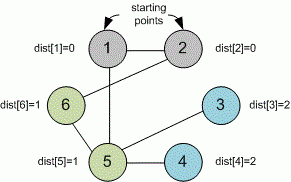Problems

# Arson

Time limit 2 seconds
Memory limit 128 MiB

There is a common web in front of you. However, as an experienced contester, you noticed that it is a connected graph with n vertices and m edges. If you fire some vertex, it will lights up, after a second all adjacent vertices light up, then all adjacent ones with already burning will light up, etc.

You know which vertices will be fired in the web (all at the same time). Find how many seconds will pass until the last vertex lights up and find this vertex.

## Input data

First line contains integers n (1n10^5) and m (n - 1m10^5). Each of the next m lines contains two numbers – the vertex numbers connected with an edge. The vertices are numbered starting from 1.

Next line contains number k (1kn) – the number of points to fire. Next line contains the numbers of k vertices to be fired.

## Output data

In the first line print the time when the last vertex will light up. In the second line print the number of this vertex. If there are several of them, print the one with minimum number.## Examples

Input example #1
6 6
1 2
2 6
1 5
5 6
3 5
4 5
2
1 2

Output example #1
2
3

Author Andrej Selivanov
Source "Five for week" 05 (2013-2014), Breadth First Search